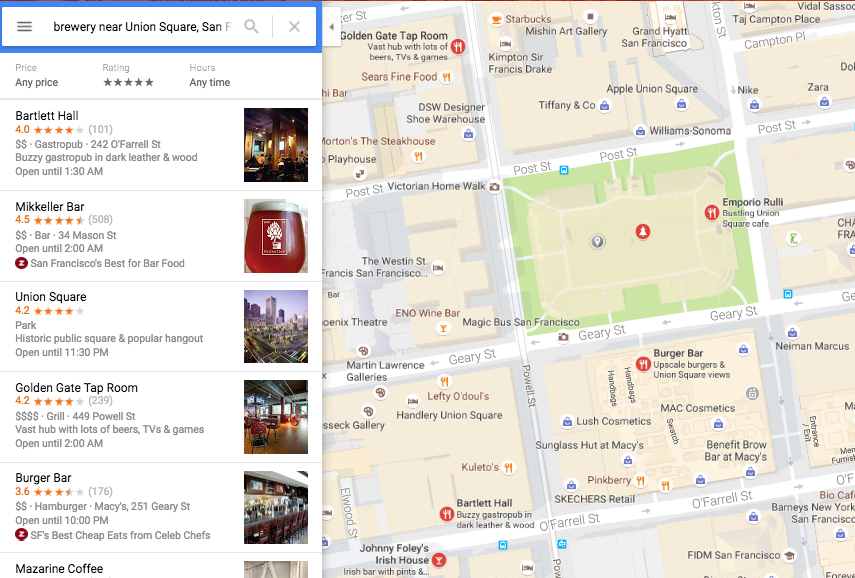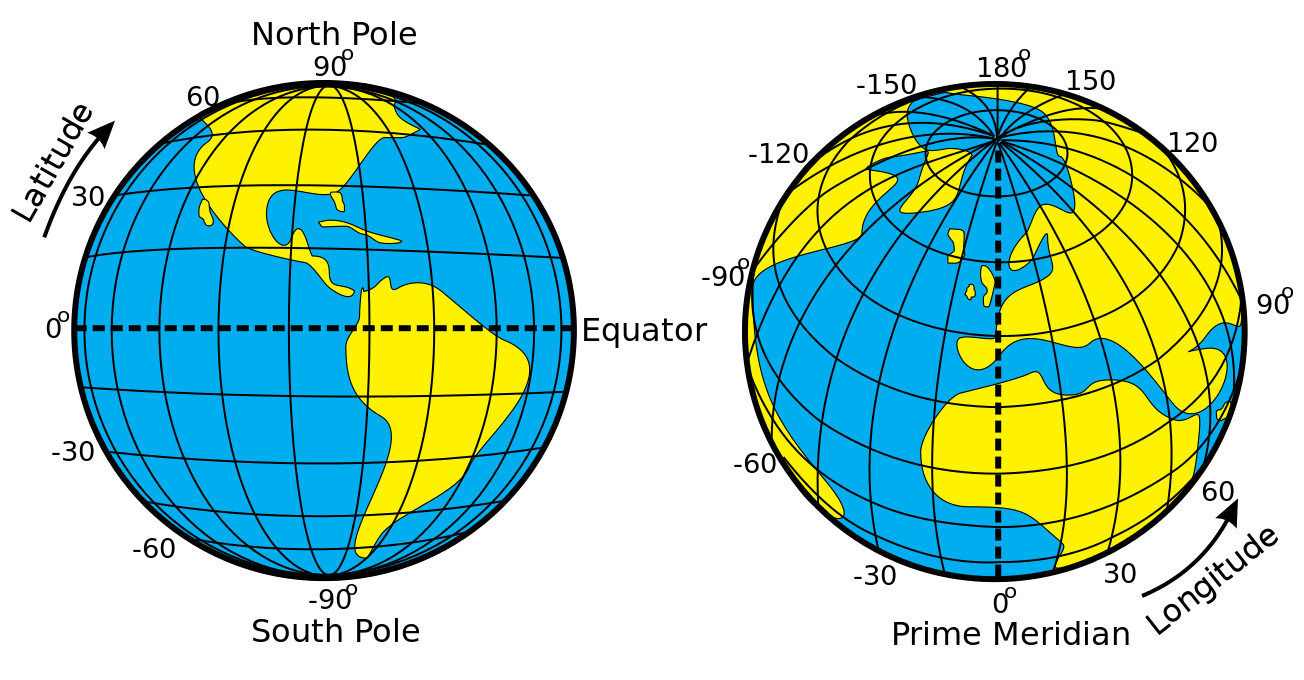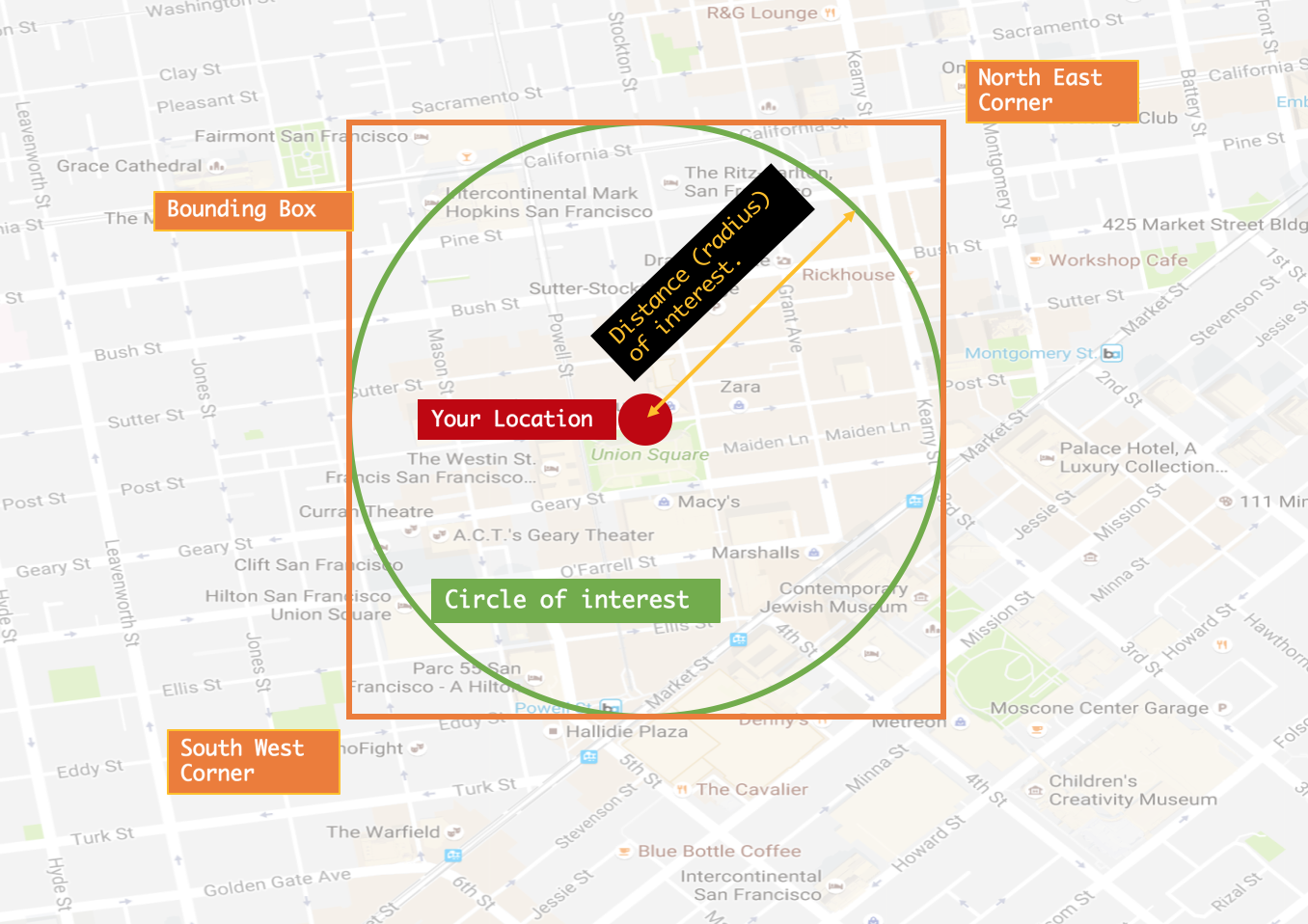## Sunday, January 8, 2017

### How to Speed Up Spatial Search in Couchbase N1QL

Location-based services like YelpUber, and Foursquare are ubiquitous. This article shows an easy way to use Couchbase N1QL to issue spatial queries and use GSI index to speed up those queries to meet your SLAs.
Whether you just finished a long SF Giants extra innings win or spent the afternoon running up and down the Lombard Street in San Francisco, sometimes, you just want a cold one, really fast. When you search on your app, you expect the answer quickly, with ratings, distance, and all. Thanks to GPStriangulation, and IP-based location detection, apps can determine your current location.
Google does this. Yelp does this. Uber does this.
How can you use your spatial data to provide location-based services or information?Here is an example of spatial data for two breweries from the beer-sample data set shipped with Couchbase.
`  {`
`    "geo": {`
`      "accuracy": "ROOFTOP",`
`      "lat": 37.7825,`
`      "lon": -122.393`
`    },`
`    "name": "21st Amendment Brewery Cafe"`
`  },`
`  {`
`    "geo": {`
`      "accuracy": "RANGE_INTERPOLATED",`
`      "lat": 50.7668,`
`      "lon": 4.3081`
`    },`
`    "name": "3 Fonteinen Brouwerij Ambachtelijke Geuzestekerij"`
`  }`
Each numerical pair, geo.lat and geo.lon represent latitude and longitude on earth. You’re in Union Square, San Francisco with latlong (37.21, -123.38). And you have a database with breweries and their locations.These are the typical questions to answer for your application.
1. Search breweries within 10 kilometers of my location.
2. Give the distances to each of these breweries.
3. Give me a sorted list of these breweries.
The application may have additional concerns about relevance, sorting by distance, rating, etc. Let's focus on finding answers to the three questions above and doing so efficiently.

## 1. Search Breweries Within 10 Kilometers of My Location

First, let's look at the sample data shipped with Couchbase.
`SELECT * FROM `beer-sample` WHERE type = 'brewery' LIMIT 1;`
`[`
`  {`
`    "beer-sample": {`
`      "address": [`
`        "563 Second Street"`
`      ],`
`      "city": "San Francisco",`
`      "code": "94107",`
`      "country": "United States",`
`      "description": "The 21st Amendment Brewery offers a variety of award winning house made brews and American grilled cuisine in a comfortable loft like setting. Join us before and after Giants baseball games in our outdoor beer garden. A great location for functions and parties in our semi-private Brewers Loft. See you soon at the 21A!",`
`      "geo": {`
`        "accuracy": "ROOFTOP",`
`        "lat": 37.7825,`
`        "lon": -122.393`
`      },`
`      "name": "21st Amendment Brewery Cafe",`
`      "phone": "1-415-369-0900",`
`      "state": "California",`
`      "type": "brewery",`
`      "updated": "2010-10-24 13:54:07",`
`      "website": "http://www.21st-amendment.com/"`
`    }`
`  }`
`]`
This is a small dataset to show full examples. The same approach described in this article will work on larger data sets as well. Bigger data sets will have bigger performance benefits!
`SELECT COUNT(*) FROM `beer-sample` WHERE type = 'brewery' ;`
`[`
`  {`
`    "\$1": 1412`
`  }`
`]`
This dataset contains a geolocation for most of the breweries. We'll be using that to find the nearest breweries.

## 2. Give the Distances to Each of These Breweries

Let's use Union Square, San Francisco with latlong (37.21, -123.38).
That's easy. simply execute this query. You'll have the results. Observe the address, city, and geo-information in the result. All are within San Francisco, with latitude and longitude pretty close to our starting point.
`SELECT country, state, city, name, geo, address `
`FROM `beer-sample` `
`WHERE (RADIANS(geo.lat) >= 0.658234696881 AND RADIANS(geo.lat) <= 0.660743280942) `
`AND (RADIANS(geo.lon) >= -2.13805724718 AND  RADIANS(geo.lon) <= -2.13488305105) `
`AND  acos(sin( RADIANS(37.7859357)) * sin (RADIANS(geo.lat)) + cos( RADIANS(37.7859357 ))  * cos(RADIANS(geo.lat)) * cos (RADIANS(geo.lon) - RADIANS( -122.4107226))) <= 0.00125568984461`
`[`
`  {`
`    "address": [`
`      "1195-A Evans Avenue"`
`    ],`
`    "city": "San Francisco",`
`    "country": "United States",`
`    "geo": {`
`      "accuracy": "RANGE_INTERPOLATED",`
`      "lat": 37.7387,`
`      "lon": -122.381`
`    },`
`    "name": "Speakeasy Ales and Lagers",`
`    "state": "California"`
`  },`
`  {`
`    "address": [`
`      "3435 Cesar Chavez #227"`
`    ],`
`    "city": "San Francisco",`
`    "country": "United States",`
`    "geo": {`
`      "accuracy": "RANGE_INTERPOLATED",`
`      "lat": 37.7481,`
`      "lon": -122.419`
`    },`
`    "name": "Shmaltz Enterprises",`
`    "state": "California"`
`  },`
`  {`
`    "address": [`
`      "1705 Mariposa Street"`
`    ],`
`    "city": "San Francisco",`
`    "country": "United States",`
`    "geo": {`
`      "accuracy": "ROOFTOP",`
`      "lat": 37.7635,`
`      "lon": -122.401`
`    },`
`    "name": "Anchor Brewing",`
`    "state": "California"`
`  },`
`  {`
`    "address": [`
`      "1398 Haight Street"`
`    ],`
`    "city": "San Francisco",`
`    "country": "United States",`
`    "geo": {`
`      "accuracy": "RANGE_INTERPOLATED",`
`      "lat": 37.7702,`
`      "lon": -122.445`
`    },`
`    "name": "Magnolia Pub and Brewery",`
`    "state": "California"`
`  },`
`  {`
`    "address": [],`
`    "city": "San Francisco",`
`    "country": "United States",`
`    "geo": {`
`      "accuracy": "APPROXIMATE",`
`      "lat": 37.7749,`
`      "lon": -122.419`
`    },`
`    "name": "Golden Gate Park Brewery",`
`    "state": "California"`
`  },`
`  {`
`    "address": [],`
`    "city": "San Francisco",`
`    "country": "United States",`
`    "geo": {`
`      "accuracy": "APPROXIMATE",`
`      "lat": 37.7749,`
`      "lon": -122.419`
`    },`
`    "name": "Shmaltz Brewing Company",`
`    "state": "California"`
`  },`
`  {`
`    "address": [`
`      "650 Fifth Street #403"`
`    ],`
`    "city": "San Francisco",`
`    "country": "United States",`
`    "geo": {`
`      "accuracy": "ROOFTOP",`
`      "lat": 37.7756,`
`      "lon": -122.398`
`    },`
`    "name": "Big Bang Brewery (Closed)",`
`    "state": "California"`
`  },`
`  {`
`    "address": [`
`      "563 Second Street"`
`    ],`
`    "city": "San Francisco",`
`    "country": "United States",`
`    "geo": {`
`      "accuracy": "ROOFTOP",`
`      "lat": 37.7825,`
`      "lon": -122.393`
`    },`
`    "name": "21st Amendment Brewery Cafe",`
`    "state": "California"`
`  },`
`  {`
`    "address": [`
`      "661 Howard Street"`
`    ],`
`    "city": "San Francisco",`
`    "country": "United States",`
`    "geo": {`
`      "accuracy": "ROOFTOP",`
`      "lat": 37.7855,`
`      "lon": -122.4`
`    },`
`    "name": "ThirstyBear Brewing",`
`    "state": "California"`
`  }`
`]`
Now, you ask, how did I come up with this gobbledygook query? What query should I run if the location is different? Those are fair questions. Let's take a detour here.
The approach and the math are described by Jan Philip Matuschek in this paper. There are several implementations of this approach to finding the bounding box for a given latlong. I used the Python implementation by Jeremy Fein to find the bounding box.
Once you have the bounding box, the paper shows you how to write the SQL query and deal with the 180th meridian problem, as well. The next step is to simply write the query in N1QL and execute it!
Here's the full implementation of this in Python.  This example python program shows you how to retrieve the results from the N1QL query.Given the location and area of interest:
• The location and distance give you the circle of interest.
• Given the same info, calculate the bounding box and determine its corner geolocation.
• Determine if this bounding box crosses 180th meridian or not.
• The N1QL query formed has two sets of predicates.
• Determine all the points within the bounding box.
• From the qualified items in the step above, filter the points falling outside the circle.
• You have the result now!
Let's look at the main portion of this.
`    # Parameters`
`    # loc = GeoLocation.from_degrees(37.21, -123.38) # Union Square, San Francisco`
`    # distance = 10  # kilometer`
`    # Get the latitude, longitude and distance`
`    lat =  float(sys.argv)`
`    lon =  float(sys.argv)`
`    distance =  float(sys.argv)`
`    # Calculate the radians.`
`    loc = GeoLocation.from_degrees(lat, lon)`
`    # Calculate the bounding box for the location and given distance`
`    SW_loc, NE_loc = loc.bounding_locations(distance)`
`    # Are we crossing the 180th meridian or not`
`    meridian180condition = (" OR " if (SW_loc.rad_lon > NE_loc.rad_lon) else " AND ")`
`    # Generate the query`
`    query = "SELECT country, state, city, name, geo FROM `beer-sample` WHERE " + \`
`    "(RADIANS(geo.lat) >= " + str(SW_loc.rad_lat) + " and RADIANS(geo.lat) <= " + str(NE_loc.rad_lat) + ") and " \`
`    "(RADIANS(geo.lon) >= " + str(SW_loc.rad_lon) +  meridian180condition + " RADIANS(geo.lon) <= " + str(NE_loc.rad_lon) + ")"  \`
`    + " AND " \`
`    " acos(sin( RADIANS(" + str(loc.deg_lat) + ")) * sin (RADIANS(geo.lat)) + cos( RADIANS(" + str(loc.deg_lat) +  " )) " \`
`    + " * cos(RADIANS(geo.lat)) * cos (RADIANS(geo.lon) - RADIANS( " + str(loc.deg_lon) + "))) <= " + str(distance/6371.0)`
`    print query`
Yes, it's that simple.
• Given the location, lat, long, and distance, calculate the bounding box.
• Execute the SQL with the expression to get the results.
Now, this query executes in about 356 milliseconds. You say, "That's too much for my customer wanting to get a cold one really quickly!" I agree. Let's look at the query plan.
`      "~children": [`
`        {`
`          "#operator": "PrimaryScan",`
`          "index": "beer_primary",`
`          "keyspace": "beer-sample",`
`          "namespace": "default",`
`          "using": "gsi"`
`        },`
PrimaryScan is never a good approach to get the best performance. As that data grows, the time taken will grow more than linearly, consuming additional network, CPU, memory resources, etc. It'll increase the latency and reduce throughput.
Let’s look at the query we used again.
`SELECT country, state, city, name, geo, address `
`FROM `beer-sample` `
`WHERE (RADIANS(geo.lat) >= 0.658234696881 `
`       AND RADIANS(geo.lat) <= 0.660743280942) `
`AND   (RADIANS(geo.lon) >= -2.13805724718 `
`       AND  RADIANS(geo.lon) <= -2.13488305105) `
`AND   acos(sin( RADIANS(37.7859357)) * sin (RADIANS(geo.lat)) + `
`       cos( RADIANS(37.7859357 ))  * cos(RADIANS(geo.lat)) * `
`       cos (RADIANS(geo.lon) - RADIANS( -122.4107226))) `
`        <= 0.00125568984461`
In this query, the first two expressions simply operate on geo.lat, geo.lon with the RADIANS() function on the values. We can create a GSI Index on it.
`CREATE INDEX idx_brewery_geo_latlon ON `
`       `beer-sample`(RADIANS(geo.lat), RADIANS(geo.lon));`
Now, let’s execute the same query again to see the performance.
`{`
`    "requestID": "8d85b9e0-1c65-4e88-835d-2e1d5a7a52b7",`
`    "signature": {`
`        "address": "json",`
`        "city": "json",`
`        "country": "json",`
`        "geo": "json",`
`        "name": "json",`
`        "state": "json"`
`    },`
`    "results": [`
`        {`
`            "address": [`
`                "1195-A Evans Avenue"`
`            ],`
`            "city": "San Francisco",`
`            "geo": {`
`                "accuracy": "RANGE_INTERPOLATED",`
`                "lat": 37.7387,`
`                "lon": -122.381`
`            },`
`            "name": "Speakeasy Ales and Lagers"`
`        },`
`….`
`    "status": "success",`
`    "metrics": {`
`        "elapsedTime": "8.341ms",`
`        "executionTime": "8.311ms",`
`        "resultCount": 9,`
`        "resultSize": 3405`
`    }`
`}`
Wow, that's 41 times improvement for this meager data set! Imagine the improvements you'll see for an even larger data set. It's like reducing a six-hour flight to eight minutes!
Again, referring back to the paper on geo locations, we know the way to calculate the distance between two geo locations:
dist = arccos(sin(lat1) · sin(lat2) + cos(lat1) · cos(lat2) · cos(lon1 - lon2)) · R
R is the radius of the earth. We simply do that calculation in the SELECT statement to calculate the distance and then orbit the distance (expression is aliased to distance in the SELECT clause).
`SELECT name, geo, address, (acos(sin( RADIANS(37.7859357)) * sin (RADIANS(geo.lat)) + `
`       cos( RADIANS(37.7859357 ))  * cos(RADIANS(geo.lat)) * `
`       cos (RADIANS(geo.lon) - RADIANS( -122.4107226))) * 6371) distance`
`FROM `beer-sample` `
`WHERE (RADIANS(geo.lat) >= 0.658234696881 `
`       AND RADIANS(geo.lat) <= 0.660743280942) `
`AND   (RADIANS(geo.lon) >= -2.13805724718 `
`       AND  RADIANS(geo.lon) <= -2.13488305105) `
`AND   acos(sin( RADIANS(37.7859357)) * sin (RADIANS(geo.lat)) + `
`       cos( RADIANS(37.7859357 ))  * cos(RADIANS(geo.lat)) * `
`       cos (RADIANS(geo.lon) - RADIANS( -122.4107226))) `
`        <= 0.00125568984461`
`ORDER BY distance asc`
`LIMIT 5;`
`[`
`  {`
`    "address": [`
`      "661 Howard Street"`
`    ],`
`    "distance": 0.9435275834385553,`
`    "geo": {`
`      "accuracy": "ROOFTOP",`
`      "lat": 37.7855,`
`      "lon": -122.4`
`    },`
`    "name": "ThirstyBear Brewing"`
`  },`
`  {`
`    "address": [],`
`    "distance": 1.426534124430217,`
`    "geo": {`
`      "accuracy": "APPROXIMATE",`
`      "lat": 37.7749,`
`      "lon": -122.419`
`    },`
`    "name": "Shmaltz Brewing Company"`
`  },`
`  {`
`    "address": [],`
`    "distance": 1.426534124430217,`
`    "geo": {`
`      "accuracy": "APPROXIMATE",`
`      "lat": 37.7749,`
`      "lon": -122.419`
`    },`
`    "name": "Golden Gate Park Brewery"`
`  },`
`  {`
`    "address": [`
`      "650 Fifth Street #403"`
`    ],`
`    "distance": 1.6034394307234021,`
`    "geo": {`
`      "accuracy": "ROOFTOP",`
`      "lat": 37.7756,`
`      "lon": -122.398`
`    },`
`    "name": "Big Bang Brewery (Closed)"`
`  },`
`  {`
`    "address": [`
`      "563 Second Street"`
`    ],`
`    "distance": 1.6036323776739865,`
`    "geo": {`
`      "accuracy": "ROOFTOP",`
`      "lat": 37.7825,`
`      "lon": -122.393`
`    },`
`    "name": "21st Amendment Brewery Cafe"`
`  }`
`]`

## 3. Give Me a Sorted List of These Breweries

The previous query will give you the qualifying nearest breweries. Now, you want additional filters like ratings, cost, etc. The additional requirements are bread and butter requirements for B-Tree indexes. Simply add new fields to your index.
`CREATE INDEX idx_beer_sample_loc_rating_cost on `beer-sample``
`    (RADIANS(geo.lat), RADIANS(geo.lon), ratings, cost) `
`    WHERE type = 'brewery';`
Now, let's look for the nearest breweries with a 4-star rating and a cost level of "medium."
`SELECT country, state, city, name, geo, address ,`
`       (acos(sin( RADIANS(37.7859357)) * sin (RADIANS(geo.lat)) +`
`           cos( RADIANS(37.7859357 ))  * cos(RADIANS(geo.lat)) *`
`           cos (RADIANS(geo.lon) - RADIANS( -122.4107226))) * 6371) AS distance`
`FROM `beer-sample``
`WHERE (RADIANS(geo.lat) >= 0.658234696881`
`       AND RADIANS(geo.lat) <= 0.660743280942)`
`AND   (RADIANS(geo.lon) >= -2.13805724718`
`       AND  RADIANS(geo.lon) <= -2.13488305105)`
`AND   acos(sin( RADIANS(37.7859357)) * sin (RADIANS(geo.lat)) +`
`       cos( RADIANS(37.7859357 ))  * cos(RADIANS(geo.lat)) *`
`       cos (RADIANS(geo.lon) - RADIANS( -122.4107226)))`
`        <= 0.00125568984461`
`AND ratings = 4`
`AND cost = 'medium'`
`AND type = 'brewery'`
`ORDER BY distance ASC;`
See the explanation below. See the span information. The index is the filtering location, rating, and cost in one big swoop! The more filtering the index does, the faster you get your results. That improves both latency, throughput, and utilization. Finally, the query simply retrieves the qualified breweries, calculates the specific distance, and sorts them. Use this technique if you need to incorporate spatial search on your app.
Maybe your boss will buy you a cold one for it!
`        {`
`          "#operator": "Sequence",`
`          "~children": [`
`            {`
`              "#operator": "IndexScan",`
`              "index": "idx_beer_sample_loc_rating_cost",`
`              "index_id": "bf5bed9f5b237493",`
`              "keyspace": "beer-sample",`
`              "namespace": "default",`
`              "spans": [`
`                {`
`                  "Range": {`
`                    "High": [`
`                      "0.660743280942",`
`                      "(-2.13488305105)",`
`                      "4",`
`                      "\"medium\""`
`                    ],`
`                    "Inclusion": 3,`
`                    "Low": [`
`                      "0.658234696881",`
`                      "(-2.13805724718)",`
`                      "4",`
`                      "\"medium\""`
`                    ]`
`                  }`
`                }`
`              ],`
`              "using": "gsi"`
`            },`

## References

### Searching JSON: comparing text search in Couchbase and MongoDB.

“Apps without search is like Google homepage without the search bar.” "Apps without search is like Google homepage withou...